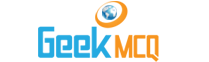# Engineering :: Economics

1.  If P represents the principal n the number of interest periods and i the interest rate based on the length of one interest period the amount of simple interest during n interest periods is
 A. P (1 + in) B. P(1 + i)n C. P i n D. P [(1 + i)n-1]

2.  If P represents the principal n the number of interest periods and i the interest rate based on the length of one interest period the amount of compounded interest during n interest periods is
 A. P (1 + in) B. P(1 + i)n C. P i n D. P [(1 + i)n-1]

3.  The nominal and effective interest rates are equal when the interest is compounded
 A. monthly B. continuously C. semi-annually D. annually

4.  If the interest rate is 5 per cent per period and the interest is compounded at half-year periods the actual annual return on the principal would be
 A. exactly 5 per cent B. exactly 10 per cent C. somewhat larger than 10 per cent D. greater than 5 per cent but less than 10 per cent

5.  Someone desires to borrow Rs. 2000 to meet his financial obligation. This money can be borrowed from a loan agency at a monthly interest rate of 3 per cent. What is the total amount of principal plus simple interest due after 3 years if no intermediate payments are made?
 A. Rs. 2180 B. Rs. 2160 C. Rs. 4160 D. Rs 20000

6.  A bound has a maturity value of Rs. 2420 and is paying discrete compound interest at an effective annual rate of 10 per cent. Determine the discount if the bond reaches maturity value in two years time.
 A. Rs. 242 B. Rs. 420 C. Rs. 2000 D. Rs 508

7.  If S represents the amount available after n interest periods for an initial pincipal P with the discrete compound interest rate i the present worth can be determined by
 A. Present worth = S/(1 + i)n B. Present worth = S/ein C. Present worth = S/(1 + in) D. Present worth = S/(1 + n)

8.  What will be the total amount available 10 years from now if Re. 1 is deposited at the present time wih nominal interest at the rate of 11 per cent compounded continuously?
 A. e110 B. 10 e0.11 C. 10 In 11 D. e1.1

9.  The effective annual interest rate ieff is expressed in terms of the nominal interest rate r compounded continuously as
 A. ieff = In (r + 1) B. ieff = er - 1 C. ieff = In r - 1 D. ieff = er

10.  For a nominal interest rate of 20 per cent compounded continuously the effective annual interest rate will be
 A. equal to 20 per cent B. more than 20 per cent C. less than 20 per cent D. equal to or less than 20 per cent